# Hypothesis Tests

 Appendix B Hypothesis Tests

## Contents

The RGA software provides two types of hypothesis tests: common beta hypothesis (CBH) and Laplace trend. Both tests are applicable to the following data types:

• Times-to-failure data
• Multiple Systems - Concurrent Operating Times
• Multiple Systems with Dates
• Multiple Systems with Event Codes
• Fielded data
• Repairable Systems
• Fleet

## Common Beta Hypothesis Test

The common beta hypothesis (CBH) tests the hypothesis that all systems in the data set have similar values of beta. As shown by Crow , suppose that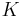number of systems are under test. Each system has an intensity function given by: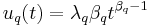where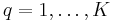. You can compare the intensity functions of each of the systems by comparing the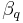of each system. When conducting an analysis of data consisting of multiple systems, you expect that each of the systems performed in a similar manner. In particular, you would expect the interarrival rate of the failures across the systems to be fairly consistent. Therefore, the CBH test evaluates the hypothesis,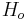, such that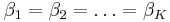. Let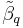denote the conditional maximum likelihood estimate of, which is given by: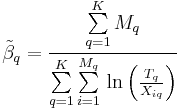where:

•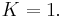•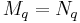if data on the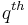system is time terminated or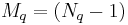if data on thesystem is failure terminated (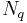is the number of failures on thesystem).
•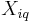is the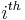time-to-failure on thesystem.

Then for each system, assume that: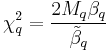are conditionally distributed as independent chi-squared random variables with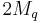degrees of freedom. When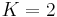, you can test the null hypothesis,, using the following statistic: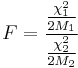Ifis true, then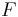equals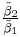and conditionally has an F-distribution with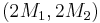degrees of freedom. The critical value,, can then be determined by referring to the chi-squared tables. Now, if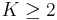, then the likelihood ratio procedure can be used to test the hypothesis, as discussed in Crow . Consider the following statistic: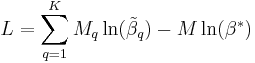where:

••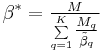Also, let: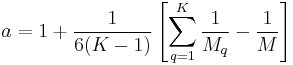Calculate the statistic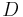, such that: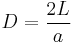The statisticis approximately distributed as a chi-squared random variable with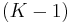degrees of freedom. Then after calculating, refer to the chi-squared tables withdegrees of freedom to determine the critical points.is true if the statisticfalls between the critical points.

### Common Beta Hypothesis Example

Consider the data in the following table.

 Repairable System Data System 1 System 2 System 3 Start 0 0 0 End 2000 2000 2000 Failures 1.2 1.4 0.3 55.6 35 32.6 72.7 46.8 33.4 111.9 65.9 241.7 121.9 181.1 396.2 303.6 712.6 444.4 326.9 1005.7 480.8 1568.4 1029.9 588.9 1913.5 1675.7 1043.9 1787.5 1136.1 1867 1288.1 1408.1 1439.4 1604.8

Given that the intensity function for thesystem is, test the hypothesis that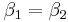while assuming a significance level equal to 0.05. Calculate the maximum likelihood estimates of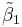and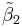. Therefore:Then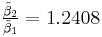. Calculate the statisticwith a significance level of 0.05.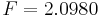Since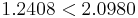we fail to reject the null hypothesis thatat the 5% significance level.

Now suppose that we test the hypothesis that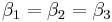. Calculate the statistic.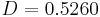Using the chi-square tables withdegrees of freedom, the critical values at the 2.5 and 97.5 percentiles are 0.1026 and 5.9915, respectively. Since, we fail to reject the null hypothesis thatat the 5% significance level.

## Laplace Trend Test

The Laplace trend test evaluates the hypothesis that a trend does not exist within the data. The Laplace trend test can determine whether the system is deteriorating, improving, or if there is no trend at all. Calculate the test statistic,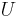, using the following equation: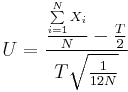where:

•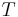= total operating time (termination time)
•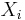= age of the system at thesuccessive failure
•= total number of failures

The test statisticis approximately a standard normal random variable. The critical value is read from the standard normal tables with a given significance level,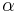.

### Laplace Trend Test Example

Consider once again the data given in the table above. Check for a trend within System 1 assuming a significance level of 0.10. Calculate the test statisticfor System 1.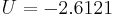From the standard normal tables with a significance level of 0.10, the critical value is equal to 1.645. If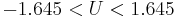then we would fail to reject the hypothesis of no trend. However, since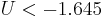then an improving trend exists within System 1. If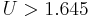then a deteriorating trend would exist.